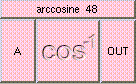ARCCOSINETHE BOX'S MAIN HELP: This function returns a scalar between [0,PI], the arccosine of A. Arccosine is the inverse of the cosine function where the acos(x) = y if and only if cos(y) = x. A (input): This must be a scalar between [-1,1]. OUT (output): This function returns a scalar between [0,PI], the arccosine of A. Arccosine is the inverse of the cosine function where the acos(x) = y if and only if cos(y) = x.

<-- BACK TO Math